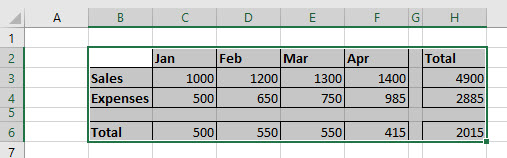# VBA Worksheet Range

This tutorial will demonstrate how to use the Worksheet Range Property in VBA.

The Worksheet.Range Propety in VBA is used to specify a cell or group of cells in Excel.  It returns the Worksheet.Range Object that represents the cell or cells and has a variety of methods available to it such as Select, Font, Copy and PasteSpecial to name but a few.

## Worksheet.Range Syntax

The syntax of the Worksheet.Range Property is simply:

``Worksheets("Sheetname").Range(Cell1,[Cell2])``

where Cell1 is required, and [Cell2] is optional.

Therefore to refer to cell A1 in sheet 1 we would type:

``Worksheets("Sheet1").Range("A1")``

To refer to cell A1 to A4 (including cells A2 and A3) in Sheet 1, we can refer to the first cell, then use a colon, and refer to the last cell in the range.

``Worksheets("Sheet1").Range("A1:A4")``

Alternatively, we can use the [Cell2] argument.  This would also include cells A2 and A3 in the worksheet range.

``Worksheets("Sheet1").Range("A1", "A4")``

If we want to refer to 2 cells that are non-contiguous, we can put a comma between the 2 cell addresses.

``Worksheets("Sheet1").Range("A1, C1")``

## Worksheet.Range Properties and Methods

Once we have used the Range Property of the Worksheet object to refer to our Range, we can then use a variety of properties and methods that are now available to the Range Object to control the appearance and /or behavior of the cells using VBA.

To see the Properties and Methods of the Range Object, we  can type a period after we have declared our range.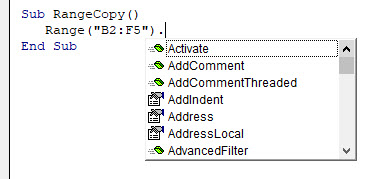Properties will show up with the small ‘hand’ icon, while methods will show up with the small green ‘block’ icon.

## Select Method

One of the most common methods to use is the select method.  This enables use to select specific cells or a cell in the Excel worksheet.

To select a single cell in a worksheet, we can just refer to the single cell in the Worksheet.Range Object

``````Sub SelectRange
Worksheets("Sheet1").Range("B2").Select
End Sub``````

To select  a range of cells, we can refer to a range of cells in the Worksheet.RangeObject.

``````Sub SelectRange
Worksheets("Sheet1").Range("B2:C5").Select
End Sub``````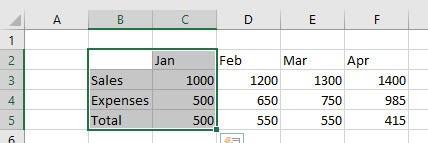To select 2 non-contiguous ranges, we  can use a comma between 2 ranges.

``````Sub SelectWorksheetRange
Worksheets("Sheet1").Range("B3:B5, C2:F2").Select
End Sub``````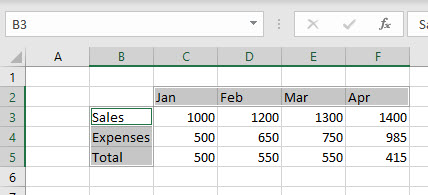## Copy and PasteSpecial Methods

We can copy the information from cells to other cells using the Copy and PasteSpecial Methods.

``````Sub WorksheetRangeCopy()
Worksheets("Sheet1").Range("B2:F5").Copy
Worksheets("Sheet1").Range("B8").PasteSpecial xlPasteAll
End Sub``````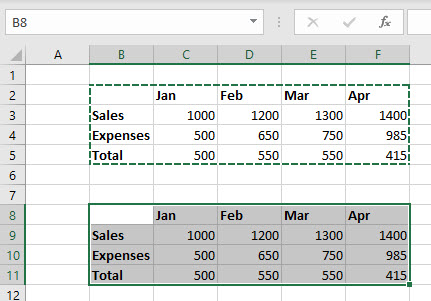## Font Property

We can use the Font Property to format the cells.  In the example below, we are using the Bold property of the Font Property to bold the cells.

``````Sub WorksheetRangeFont()
Worksheets("Sheet1").Range("B3:B5, C2:F2").Font.Bold = True
End Sub``````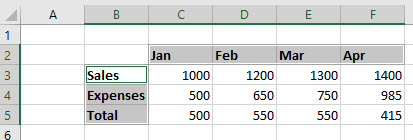## Borders Property

We can use the Borders Property, and then the LineStyle property of the Borders property to put borders around all the cells.

``````Sub WorksheetRangeCopy()
Worksheets("Sheet1").Range("B2:F5").Borders.LineStyle = xlContinuous
End Sub``````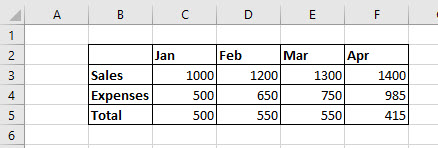## VBA Coding Made Easy

Stop searching for VBA code online. Learn more about AutoMacro - A VBA Code Builder that allows beginners to code procedures from scratch with minimal coding knowledge and with many time-saving features for all users!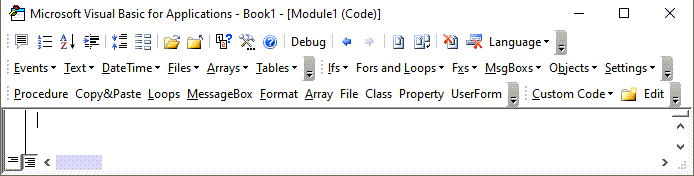## CurrentRegion Property

We can use the Current Region property of the Range object, and then the Select Method of the Current Region object to select all cells that are within the current region of the specified cell.

The current Region will select all the cells that are adjacent to the cell that is specified in the Range as long as those cells are contiguous.  It will not select any cells after a blank row or column.

``````Sub CurrentRegion()
Worksheets("Sheet1").Range("B2").CurrentRegion.Select
End Sub``````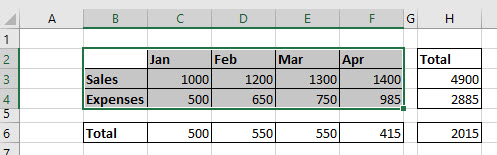## UsedRange Property

If we want to select all the cells that are populated in a worksheet, we need to use a Property of the Worksheet itself called UsedRange.

``````Sub UsedRangeSelect()
Worksheets("Sheet1").UsedRange.Select
End Sub``````